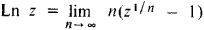# Logarithmic Function

Also found in: Dictionary, Thesaurus, Medical, Wikipedia.
The following article is from The Great Soviet Encyclopedia (1979). It might be outdated or ideologically biased.

## Logarithmic Function

a function that is the inverse of the exponential function and denoted by

(1)y = In x

Its value y, which corresponds to the value of the independent variable x, is called the natural logarithm of the number x. By definition, the relationship (1) is equivalent to

(2)x = ey

where e is the base of the natural logarithm system. Since ey > 0 for any real y, the logarithmic function is defined only for x > 0. In its most general meaning, the logarithmic function is the function

y = logax

where a > 0 (a ≠ 1) is an arbitrary logarithmic base. However, in mathematical analysis the function In x is of particular importance. The function logax can be reduced to it by the formula

loga x = M In x

where M = 1/ln a.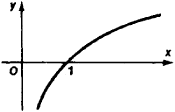Figure 1

The logarithmic function is one of the principal fundamental elementary functions. Its graph (Figure 1) is called the logarithmic curve. The basic properties of the logarithmic function follow from the corresponding properties of the exponential function and logarithms. For example, the logarithmic function satisfies the functional equation

In x + In y = I nxy

When – 1 < x ≤ 1, the logarithmic function can be expanded into a power series, that is,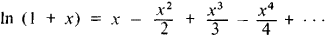Many integrals are expressed in terms of logarithmic functions; for example,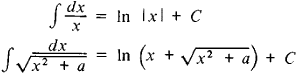The logarithmic function is common in mathematical analysis and its applications.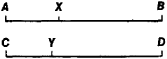Figure 2

The logarithmic function was well known to 17th-century mathematicians. J. Napier (1614) was the first to examine the connection between variable values that could be expressed by a logarithmic function. He represented such a connection between numbers and their logarithms by means of two points moving along parallel lines (Figure 2). One of them (Y) moves with constant velocity from C, while the other (X) begins its motion at A and moves with a velocity proportional to its distance from B. If we let CY = y and XB = x, then, according to this definition, dx/dy = –kx, so that y = — (1/k) In x.

The logarithmic function in the complex plane is a multiple-valued (infinite-valued) function defined for all values of an argument z ≠ 0 and denoted by Ln z. The single-valued branch of this function which can be defined by

ln z = ln ǀzǀ + i arg z

where arg z is the argument of the complex number z, is called the principal value of the logarithmic function. We have

Ln z = In z + 2kπi, k = 0, ±1, ±2, . . .

All the values of the logarithmic function for negative real Z are complex numbers. The first satisfactory theory of the logarithmic function in the complex plane was given by L. Euler (1749), who proceeded from the definition